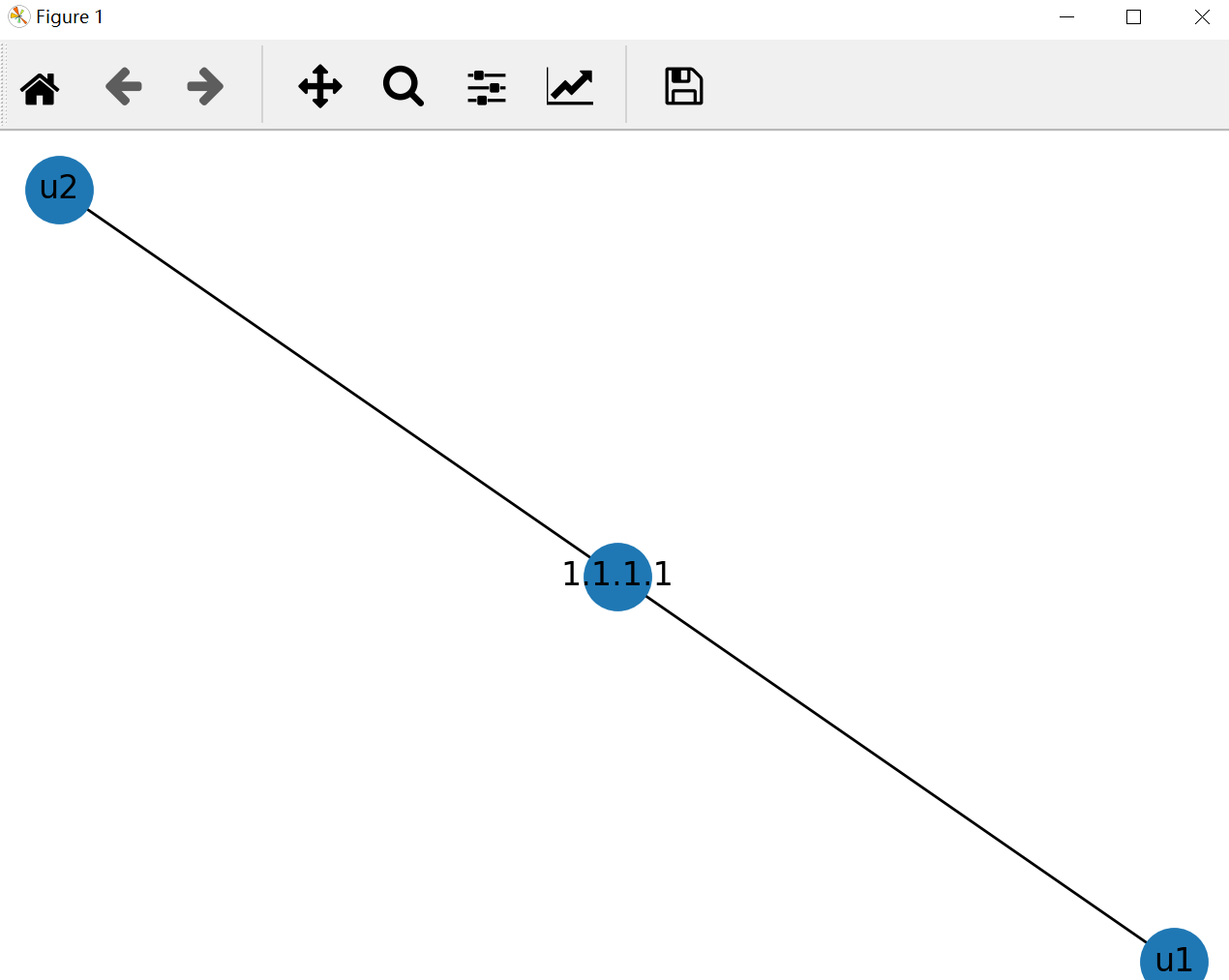# 《Web安全之机器学习入门》笔记：第十三章 13.5 知识图谱 hello word``````import networkx as nx
import matplotlib.pyplot as plt

def helloWord():
G = nx.Graph()
nx.draw(G,with_labels=True,node_size=600)
plt.show()

if __name__ == '__main__':
print ("Knowledge Graph")
helloWord()``````-- 展开阅读全文 --

Web安全—逻辑越权漏洞（BAC）
« 上一篇 03-13
Redis底层数据结构--简单动态字符串